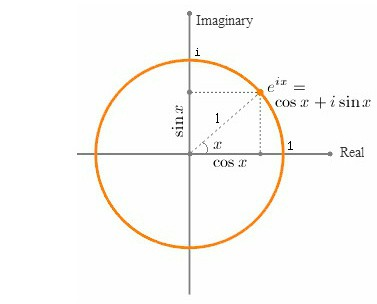## What is Euler’s Secret Identity?Is there some connection between the Mathematics of Euler’s Identity and the Physics of a Photon?

Euler’s Formula (eix = cos(x) + i sin(x) ) is usually thought of as a mathematical description of how a “vector rotates” through an angle in the complex plane; and consequently Euler’s Identity is usually considered simply a rotation through an angle of π radians.  But as long ago as 1581 Galileo had already realized something amazing; “rotation” has a secret identity — he is also an “oscillation”…

Galileo’s startling realization that uniform circular motion and periodic motion were different sides of the same coin, meant that he saw that angles could also be used to describe an oscillation.  And this, it turns out, was a truly brilliant insight!…

So although most people would probably still associate angles with geometry and trigonometry, angles can also be associated with the amount of “energy in an oscillation” –-  which means that a function that defines an amount of  rotation in the complex-plane ( y=(-1+i0))  can also be thought of as a “definable angular frequency” expressed as x% of (-1+i0), or in other words: “ x% of a half-cycle”.

Now, what is really interesting about this idea is that:  the amplitude of all “normalized” oscillations have a magnitude of 0.25 units of measurement, or ¼ of the normalized cycle.  So

• In our formula ( y=(-1+i0)), when we set  x=0.5, we get “a quarter-rotation in the complex plane”

(-1+i0)0.5   =   (0+i1)

• But in setting x=0.5 we are also, in effect, setting an angular frequency of π/2 radians for the equivalent oscillation on the real-line; and so we could also say that (0+i1) is “a quarter-oscillation on the real-line”, which means that

(0+i) is the amplitude of all normalized oscillations

• And this means that

(-1) is the “amplitude of an normalized oscillation squared (i2)”

• And this means that

all frequencies can be defined as some % of (i2)

=======

So, any angular frequency of oscillation, defined in terms of a single half-cycle ( y = (-1)x ), can now also be defined in terms of the normalized amplitude squared  ( y = (i2)x ).  And this, to anyone who has studied even a small amount of physics is strangely reminiscent of the “energy in simple harmonic motion”.

This would seem to suggest that an angular frequency (i2)x  might be equivalent is some way to the energy in an oscillation (½kA2), and that (x) might represent some form of “restoring variable”.

Furthermore since we know that the equation for the energy in a photon of light is

E  =  hf  =  ħω

This further suggests that there might be some form of a connection between (eiπx) and (ħω).  So let’s take a closer look at ( ei )…

We can use Euler’s Formula to define a smallest “unit of angular change”.  Let’s define this unit to have the same magnitude as the value of Planck’s Reduced Constant.eix   =   =   cos(x) +  i sin(x)

ei   =   cos(1) +  i sin(1)

eiħ   =   cos(ħ) +  i sin(ħ)

(-1)ħ   =   (0.99999999 +  iħ)

Given this defined “unit of angular change”, we can now think of every other size of angular change as an integer number(n) of units of (1 +iħ);

(eiħ)n   =   (ei)ħn   =   einħ   =   (1 + iħ)n   =   n ħ-radians   (where n = 1,2,3….)

And when n = Ħ =  9.48252238653324  x  10+33 (i.e. capital-h-bar : the inverse of  ħ) we get an angle of

(eiħ)Ħ   =   (ei)ħĦ   =   ei   =   (1 + iħ)Ħ   =   Ħ ħ-radians =   1 radian

And when n = Ħπ we get an angle of

(eiħ)Ħπ   =   (ei)ħĦπ   =   eiπ   =   (1 + iħ)Ħπ   =   π radians  = (-1+i0)So if (-1)x  is indeed equivalent to  (½kA2) we could now say that since

(-1)x   =   (eiπ)x   =   (1+ iħ) Ħπx

And since

½kA2   =   hf

ThereforeSo maybe different photon frequencies simply represent different amounts of restoring force in the elastic fabric of space-time.  If so then different frequencies would simply represent different percentages of the ultimate elasticity of space-time.

So what is Euler’s Secret Identity?

Maybe he is the speed of light’s cousin;

“The Angular Speed of Mass” – the fastest frequency of all…

Posted on Categories Uncategorized